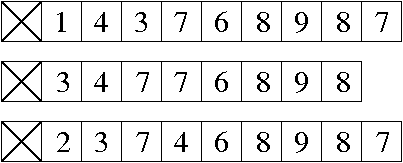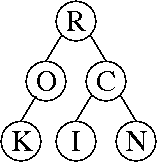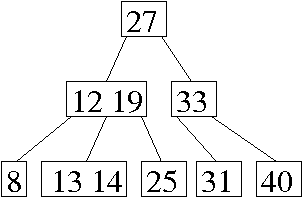CS 61B: Data Structures (Spring 2009)
Midterm II

### Solutions

Problem 1. (12 points) A Miscellany.

a.b. BException.

c. Alpha-beta search prunes nodes N, H, Q, and R.

d. 999. (Note that even if G contains self-edges, breadth-first search never creates a self-edge in T, because it never visits a node twice.)

e. 1.

f. No.

Because f(n) ∈ O(g(n)), there exist positive constants c and N such that f(n) ≤ c g(n) for all n > N.

Because h(n) ∈ &Omega(ng(n)), there exist positive constants d and P such that h(n) ≥ d n g(n) for all n > P.

Suppose that f(n) ∈ Θ(h(n)). Then there exist positive constants e and M such that f(n) ≥ e h(n) for all n > M.

Putting these three together, we have that f(n) ≥ ed n g(n) ≥ (ed / c) n f(n) for all n > max{M, N, P}.

In other words, nc / (ed) for all n > max{M, N, P}, which is impossible.

Problem 2. (6 points) Trees.

a.b. Θ(r log (n + r)). This can also be written Θ(r log n + r log r)).

c.d. Oops! I wrote “ith-largest”, but I meant to write “ith-smallest”, so I made the problem even harder than necessary. Below are two algorithms that find the ith-smallest key. To find the ith-largest key, just replace i with n + 1 – i.

Algorithm 1. We start at the root, then walk down the tree until we reach the node with the ith-smallest key. The question is, how do you know when to go left and when to go right? Since the tree is perfectly balanced, and every subtree is perfectly balanced, we can adapt binary search for this purpose. For example, if n = 15, we know the the tree contains the 1st through 15th smallest keys, so we set left &larr 1 and right &larr 15. The root is exactly in the middle, so it contains the mid &larr (left + right) / 2 = 8th smallest key.

If i = mid, we want the root key, so we're done. If i < mid, we recurse on the left subtree by setting rightmid – 1. If i > mid, we recurse on the right subtree by setting leftmid + 1. Either way, then we set mid &larr (left + right) / 2 again. Repeat until i = mid.

As we go down the tree, we must also keep track of the level number of the node we're visiting, so that when we reach the node containing the ith-smallest key, we know where to find the key in the array. We do this with the standard rules for level numbering: at the root, set x &larr 1. When we go to a left child, set x &larr 2x. When we go to a right child, set x &larr 2x + 1. When we finish, return the key at index x.

This algorithm takes Θ(log n) time. For completeness, here's code (though we didn't expect code on the exam).

```
// Find the ith largest key in a perfectly balanced BST stored by
// level numbering in array a.  a.length must be a power of 2.
// No item is stored at a.  i must be in [1, a.length - 1].
public static int ithsmallest(int[] a, int i) {
return ithsmallest(a, 1, a.length, i, 1);
}

private static int ithsmallest(int[] a, int left, int right, int i, int x) {
int mid = (left + right) / 2;            // Halfway between left and right.
if (i == mid) {
return a[x];
} else if (i < mid) {
return ithsmallest(a, left, mid - 1, i, 2 * x);
} else {
return ithsmallest(a, mid + 1, right, i, 2 * x + 1);
}
}
```
Observe that the algorithm never actually looks in the array of keys until it reaches the node containing the ith key. Until then, it is just computing indices (the inorder number and level number of the node it's visiting).

Algorithm 2. This algorithm is shorter and quicker, but it takes a lot more cleverness to think of. Whereas Algorithm 1 searches from the root down, this one searches from the leaves up.

If i is odd, then the ith-smallest key is stored in a leaf, and its array index is (n + i) / 2.

If i is even, but i / 2 is odd, then the ith-smallest key has height 1, and its array index is ⌊(n + i) / 4⌋.

If i and i / 2 are even, but i / 4 is odd, then the ith-smallest key has height 2, and its array index is ⌊(n + i) / 8⌋.

And so on. So here's one working algorithm: set ji, and set x ← (n + i) / 2. While j is even, divide both j and x by two. (Store x as an integer, so the division rounds down.) When j is odd, return the key stored at index x. This algorithm takes Θ(log n) time.

Problem 3. (7 points) Graph Adjacency Running Times.

 insert(u, v) or remove(u, v) member(u, v) printList(u) Adjacency matrix Θ(1) Θ(1) Θ(n) Sorted arrays Θ(d) Θ(log d) Θ(d) Balanced search trees Θ(log d) Θ(log d) Θ(d) One sorted array Θ(e) Θ(log e) Θ(d + log e) Dictionary Θ(1) Θ(1) Θ(e)

Mail inquiries to cs61b@cory.eecs